Next: Jeffries Connection Formula Up: Wave Propagation in Inhomogeneous Previous: Stokes Constants

# WKB Reflection Coefficient

Let us write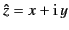, where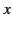and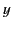are real variables. Consider the solution of the differential equation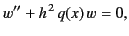(1196)

where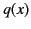is a real function,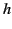is a large number,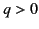for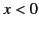, and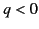for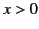. It is clear that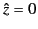represents a simple zero of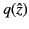. Here, we assume, as seems eminently reasonable, that we can find a well-behaved function of the complex variablesuch that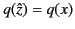along the real axis. The arrangement of Stokes and anti-Stokes lines in the immediate vicinity of the pointis sketched in Figure 24. The argument ofon the positive-axis is chosen to be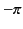. Thus, the argument ofon the negative-axis is 0 .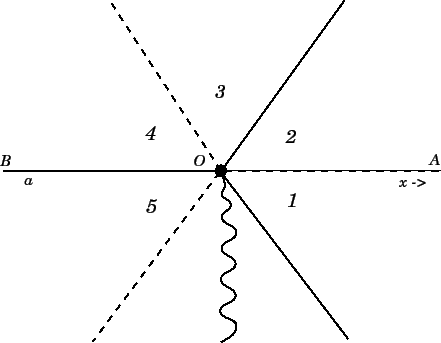On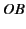, the two WKB solutions (1176)-(1177) can be written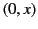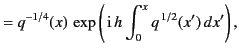(1197)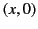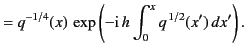(1198)

Here, we can interpretas a wave propagating to the right along the-axis, and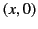as a wave propagating to the left. On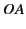, the WKB solutions take the form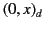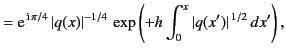(1199)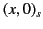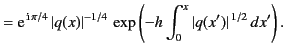(1200)

Clearly,represents an evanescent wave that decays to the right along the-axis, whereas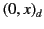represents an evanescent wave that decays to the left. If we adopt the boundary condition that there is no incident wave from the region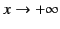, the most general asymptotic solution to Equation (1198) onis written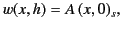(1201)

whereis an arbitrary constant.

Let us assume that we can find an analytic solution,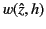, to the differential equation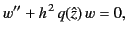(1202)

which satisfies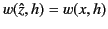along the real axis, where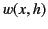is the physical solution. From a mathematical point of view, this seems eminently reasonable. In the domains 1 and 2, the solution (1203) becomes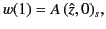(1203)

and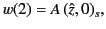(1204)

respectively. Note that the solution is continuous across the Stokes line, because the coefficient of the dominant solution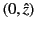is zero: thus, the jump in the coefficient of the subdominant solution is zero times the Stokes constant,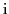. In other words, it is zero. Let us move into domain 3. In doing so, we cross an anti-Stokes line, so the solution becomes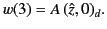(1205)

Let us now move into domain 4. In doing so, we cross a Stokes line. Applying the general rule derived in the preceding section, the solution becomes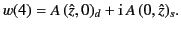(1206)

Finally, onthe solution becomes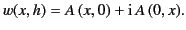(1207)

Suppose that there is a point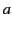on the negative-axis where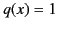. It follows from Equations (1201) and (1209) that we can write the asymptotic solution to Equation (1198) as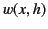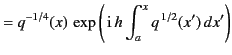(1208)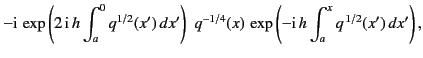in the region, and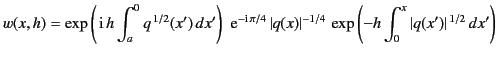(1209)

in the region. Here, we have chosen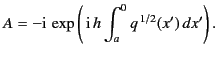(1210)

If we interpretas a normalized altitude in the ionosphere,as the square of the refractive index in the ionosphere, the pointas ground level, and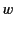as the electric field strength of a radio wave propagating vertically upwards into the ionosphere, then Equation (1210) tells us that a unit amplitude wave fired vertically upwards from ground level into the ionosphere is reflected at the level where the refractive index is zero. The first term in Equation (1210) is the incident wave, and the second term is the reflected wave. The reflection coefficient (i.e., the ratio of the reflected to the incident wave at ground level) is given by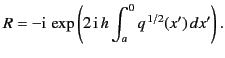(1211)

Note that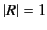, so the amplitude of the reflected wave equals that of the incident wave. In other words, there is no absorption of the wave at the level of reflection. The phase shift of the reflected wave at ground level, with respect to that of the incident wave, is that associated with the wave propagating from ground level to the reflection level and back to ground level again, plus a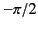phase shift at reflection. According to Equation (1211), the wave attenuates fairly rapidly (in the space of a few wavelengths) above the reflection level. Of course, Equation (1213) is completely equivalent to Equation (1089).

Note that the reflection of the incident wave at the point where the refractive index is zero is directly associated with the Stokes phenomenon. Without the jump in the coefficient of the subdominant solution, as we go from domain 3 to domain 4, there is no reflected wave on theaxis. Note, also, that the WKB solutions (1210) and (1211) break down in the immediate vicinity of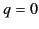(i.e., at the reflection point). Thus, it is possible to demonstrate that the incident wave is totally reflected at the point, with aphase shift, without having to solve for the wave structure in the immediate vicinity of the reflection point. This demonstrates that the reflection of the incident wave atis an intrinsic property of the WKB solutions, and does not depend on the detailed behavior of the wave in the region where the WKB solutions break down.Next: Jeffries Connection Formula Up: Wave Propagation in Inhomogeneous Previous: Stokes Constants
Richard Fitzpatrick 2014-06-27